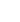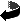# Index of /misc/stack-caching-dataNameLast modifiedSizeDescriptionParent Directory  -res-compile.gz28-Mar-1995 13:31 12Kres-cross.gz28-Mar-1995 13:31 12Kres-gray.gz28-Mar-1995 13:31 12Kres-prims2x.gz28-Mar-1995 13:31 12K

Raw data about stack caching

# Raw data about stack caching

This directory contains most of the raw empirical data for the paper Stack Caching for Interpreters by M. Anton Ertl, which appears in the SIGPLAN PLDI '95 proceedings.

The res-* files contain the data for the programs that were measured. Each file starts with a list of the execution frequencies of the primitives. Then (starting with "NEXT") comes a list of basic data like the number of instructions executed (NEXT), stack accesses without any caching in registers and with keeping the Top-of-stack in a register (1 reg), stack pointer updates, similar data for the return stack, some data for consistency checking of the measurement process (e.g., "in!=out" should occur the same number of times as "sp updates"), the number of stack manipulation words, and the number of cache resets in static stack caching.

Note that the number of stores is smaller than the number of fetches, because some of the stores are optimized away (e.g., "dup" can be describes as popping one value and pushing two, but actually only the second value need be stored). This optimization was not considered for the simulation of the stack cache. However, this should not make much difference, because this optimization only has a significant effect when no values are kept in registers: Note how small the difference is beetwen the fetches and stores for the "1 reg" case. Similarly, some of the fetches are optimized away by the dead code elimination of the C compiler (e.g., "over" reads two values, but actually does not use one of them). The data measured here does not reflect this, since we counted the fetches at the C level. The difference in the number of "stack in" and "stack out" (which is the same as the number of loads and stores respectively in the simulations) is due to the fact that the virtual machine is entered with three items on the stack and typically left with no items on the stack.

Also, the primitive "reset-state" would be produced only for static stack caching (it resets the cache to the canonical state before a control flow join). So the basic number of instructions executed is the number of NEXTs minus the number of reset-states.

Next, there's the data for keeping a constant number of registers in registers. For each number of registers there's the number of loads of stack data from memory, stores of stack data to memory, moves between the stack cache registers, and stack pointer updates. Note that the number of moves does not include the moves necessary to perform stack manipulation words.

Then, there's the data for dynamic stack caching, for different numbers of register, and for varying overflow follow-up states. Again, you have loads, stores, moves, and updates.

Finally, there's the data for static stack caching. "overflow to" here means the canonical state, which also served as the overflow followup state. In addition to the usual data, there's a column for false nexts, which indicates the number of stack manipulation instructions that can be optimized away (to be subtracted from the number of NEXTs).

You may wonder how the number of overflows was produced for the paper: It was derived from the present data by dividing the number of stores by the number of stores expected by overflow. In awk: \$9/(\$2+1-\$6). The number of stores expected is a bit of a problem: It assumes that the overflow is only by one item and it also does not work for overflow states with 0-2 registers. The assumption about overflows seems to hold for the programs except gray for the cases we looked at; and you have to ignore the results for overflow states with 0-2 registers. It would surely be better to measure the overflows explicitely. Well, next time.

If you have any further questions, mail me at anton@mips.complang.tuwien.ac.at.